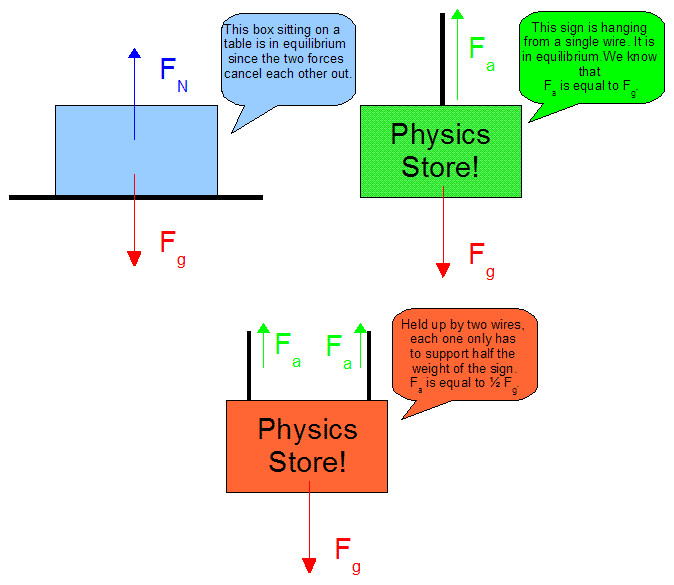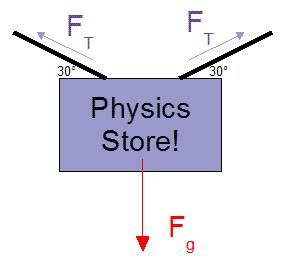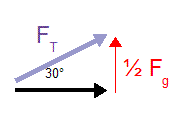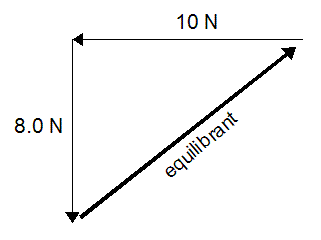Lesson 24: Equilibrium

• Equilibrium is any situation where the net force acting on an object is zero.
• We call it equilibrium because all the forces acting on the object equal out and cancel each other.
• Some situations of equilibrium are easy, others are more difficult, as the following images show.Figure 1

Each of these situations shown in Figure 1 could be solved by you back in "Net Forces", but we can also do problems that you will have to use vectors for.Example 1: A 10 kg sign is being held up by two wires that each make a 30° angle with the ground. Determine the tension (force) in each of the wires.

The diagram to the right shows what this would look like. The tension is a force in the wires that acts along the length of the wires.

• Basically, these wires are wasting some of their effort by pulling sideways against each other.
• The only force that the sign actually needs to hold it up is a force pulling up.
• Since everything else is equal, we can assume that each wire is holding up half of the weight of the sign.
• We can do our calculations for one wire, and then say that the other wire is the same (since they are just mirror images of each other).Fg = mg
= (10) (9.81)
Fg = 98.1 N

sinΘ = opp / hyp
sinΘ = 1/2 Fg / FT
FT = (1/2 Fg) / sinΘ
= (49.05) / sin 30° = 98.1 N

The tension in each wire of the sign is 98 N [30° from the horizontal].

In some situations you may already have a couple of forces acting on an object and want to know what third force would cancel them out.

• This third force that would do the cancelling out is called the equilibrant.
• The equilibrant is a vector that is the exact same size as the resultant would be, but the equilibrant points in exactly the opposite direction.
• For this reason, an equilibrant touches the other vectors head-to-tail like any other vector being added.

Example 2: Two forces are pushing an object along the ground. One force is 10 N [W] and the other is 8.0 N [S]. Sketch a diagram showing the equilibrant of these two forces, and determine the equilibrant.If you look at the sketch you'll see that the equilibrant is the exact opposite of what you would draw for a resultant.

The equilibrant takes you right back to where you started. The net force... zero!

It would almost be the same if you went for a walk and followed this as a path. You'd end up back where you started and your displacement would be zero.

Calculate the equilibrant...
c2 = a2 + b2 = 102 + 82
c = 13 N

Calculate the angle where the 8.0 N force and the equilibrant touch...
tanΘ = opp/adj = 10 / 8
Θ = 51°

The equilibrant is 13 N [N51°E].